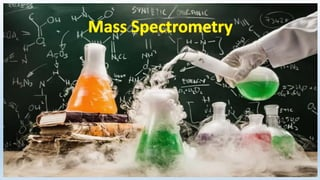Successfully reported this slideshow.×
1 of 22

Mass spectrometry

1

Share

Mass Spectrometry
it is very useful technique for the measurement of masses of isotopes, relative abundance of isotopes, molecular masses of the compounds and molecular structure.
Principle
Aston's mass spectrometer
Dempster's mass spectrometer
WOKING
Comparison with C-12

See all

See all

Mass spectrometry

1. 1. Prof. Sohaib Hasnain Punjab Group of Colleges, Faisalabad CHEMISTRY
2. 2. MASS SPECTROMETRY IS AN ANALYTICAL TECHNIQUE WHICH USED TO MEASURE:  EXACT MASSES OF ISOTOPES  RELATIVE ABUNDANCE OF IOSTOPES  MOLECULAR MASSES OF COMPOUNDS  MOLECULAR STRUCTURE
3. 3. WHAT IS MASS SPECTROMETER/ SPECTROGRAPH? Mass Spectrometer Is An Instrument Which Is Used To Measure The Exact Masses Of Different Isotopes Of An Element.
4. 4. Aston’s Mass Spectrograph • First Mass spectrograph was designed in 1919 • Used to identify the isotopes of an element on the basis of their atomic masses • Aston was awarded with noble prize for the discovery of isotopes
5. 5. Dempster’s Mass Spectrometer • Develped by A.J.Dempster in1918 • Used for the identification of elements which available in solid state
6. 6. PRINCIPLE OF MASS SPECTROMETRY In this technique, a substance is first volatilized and then ionized with the help of high energy beam of electrons. The gaseous positive ions, thus formed, are separated on the basis of their mass to charge ratio (m/e) and then recorded in the form of peaks.
7. 7. Construction And Working Of Dempster’s Mass Spectrometer Determination Of Exact Masses And Relative Abundance Of Isotopes
8. 8. MASS SPECTROMETER
9. 9. STEPS INVOLVED DURING THE WORKING: Vaporization Ionization Acceleration Deflection Collection
10. 10. Vaporization/ Volatilization • The substance whose analysis for the separation of isotopes is required, is converted into vapor phase • Vaporization is done in vaporizing chamber • The pressure kept for this step is very low 10-6 to 10-7 torr • Solid and liquid are changed into vapor phase • Vaporization is not required for the gases because these are already present in vapor phase
11. 11. Ionization • Vapor are allowed to enter in ionization chamber • Vapors are ionized with the help of high energy electrons • These positively charged ions of isotopes of an element have different masses depending upon the nature of isotope
12. 12. Ionization • We have sample of hydrogen for ionization • When high energy electrons are thrown on hydrogen atoms, its electron are knocked out • Uni positive cation are formed because only one electron is removed from its each atom • Masses of ions are different because isotopes of hydrogen have different mass • That’s why these have differet m/e Value
13. 13. Acceleration • This step is required to speed up the movement of cations • Cations are speed up by providing electric field E= 500-2000 volts • Greater the strength of electric field, greater will be the speed of cations toward the negative Grid • Now, Beam of cation is formed which is travelled in straight line
14. 14. Deflection • The beam of accelerated positive ions are allowed to pass through the magnetic field • Magnetic field is perpendicular to the direction of positive ions • Positive ion are separated due to deflection of magnetic field • This separation is based on m/e ratio • Greater the value of m/e, lower will be the deflection • lower the value of m/e, greater will be the deflection • Heavy ions are slightly deflected and Vice versa
15. 15. MASS SPECTROMETER
16. 16. Deflection Mathematical Expression m/e = H2r/E H = strength of magnetic field E = strength of electric field R = radius of circular path Larger the m/e value, larger will be the radius Greater the value of H, Smaller will the radius Greater the value of E, larger will be the radius
17. 17. Collection • This process is done by Detector, Electrometer and ion collector • Electrometer develops the electric current • The strength of current measured gives the relative abundance
18. 18. Comparison with C-12
19. 19. Mass Spectrum A Plot Of Data In Such A Way That M/E Of Isotopes Of An Element Are Taken On Abscissa (X-axis) And Relative Abundance Of Ions On Ordinate (Y-axis)